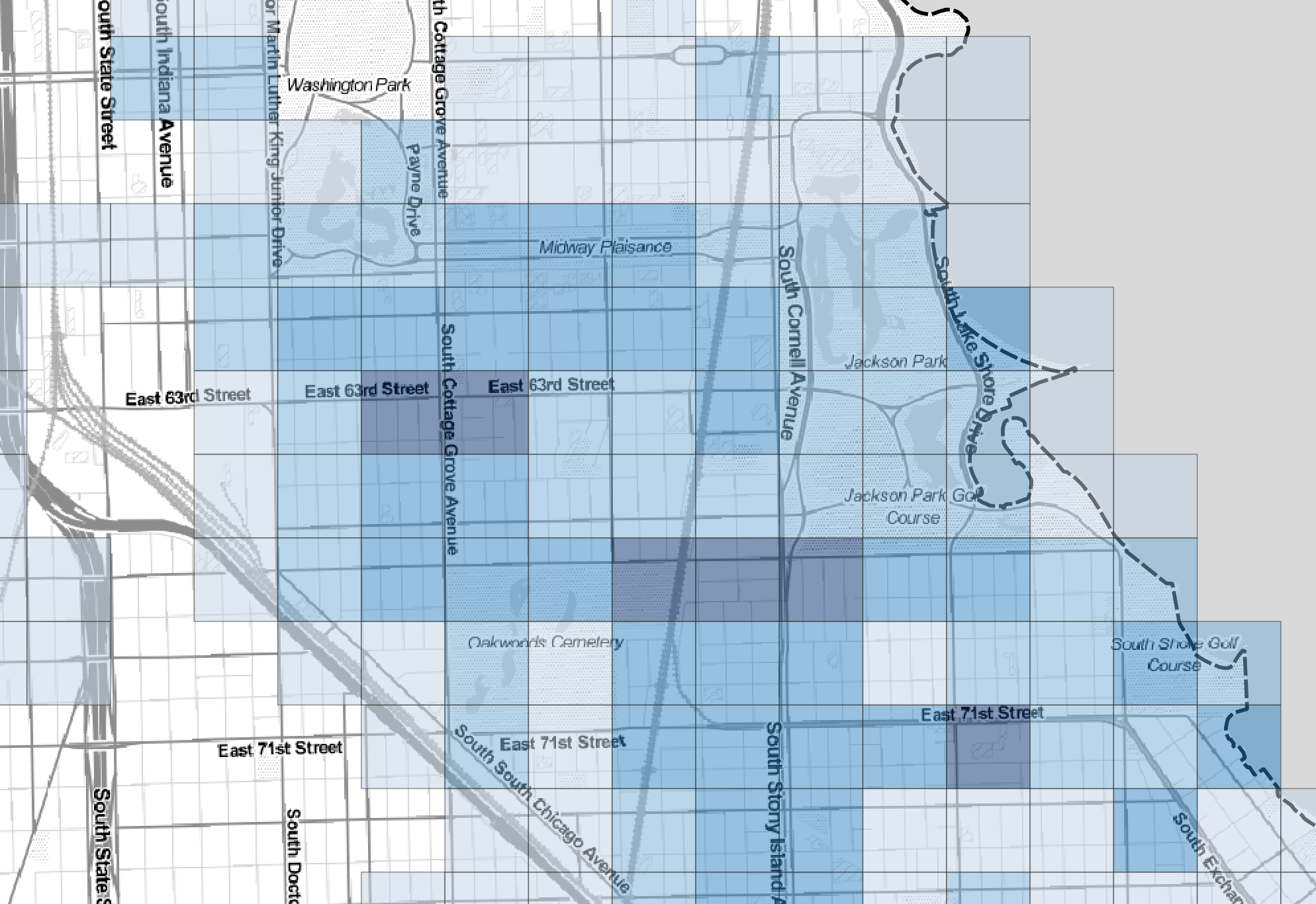A common way to show the distribution of places (like grocery stores) is to use a heat map. The map will appear “hotter” where there are many grocery stores and “colder” where there are few grocery stores. This kind of map can be useful to show gaps in distribution or a neighborhood that has a lot of grocery stores.

One issue with that kind of heat map is that the coverage areas change their shape and color if you zoom in, since the algorithm that clusters or determines what’s “nearby” or dense has fewer locations to analyze.

I prefer to use grids in the shape of square tiles, since Chicago is grid-oriented city and the vast majority of our streets and our routes move along east-west and north-south lines. The map above shows the location of subjects and topics of news articles in the Chicago Cityscape database.

I use PostGIS to set up most of my spatial data before visualizing it in QGIS.

This tutorial shows the two steps to using PostGIS to (1) create a grid based on an existing area (polygon), (2) assigning each point location to a tile in that grid and counting the number of locations in that tile.

If you don’t have PostGIS installed, you should install it if you work with spatial data a lot. It is much, much faster at performing most of the tasks you use QGIS or ArcGIS to perform. Both QGIS and ArcGIS can read and edit data stored in PostGIS.

Additionally, there is a function within QGIS that can create grids, and another function that can do comparisons by location and count/summarize, so all of this can be done without PostGIS.

For this tutorial, you will need a single polygon with which to create the grid. I used the boundary of the City of Chicago limits.

1. Create a grid based on an existing area

1.a. Add a new function to PostGIS

To create a grid, you need a function that draws the tiles based on the polygon. I got this from The Spatial Database Advisor.

``````-- Create required type
DROP   TYPE IF EXISTS T_Grid CASCADE;
CREATE TYPE T_Grid AS (
gcol  int4,
grow  int4,
geom geometry
);
-- Drop function is exists
DROP FUNCTION IF EXISTS ST_RegularGrid(geometry, NUMERIC, NUMERIC, BOOLEAN);
-- Now create the function
CREATE OR REPLACE FUNCTION ST_RegularGrid(p_geometry   geometry,
p_TileSizeX  NUMERIC,
p_TileSizeY  NUMERIC,
p_point      BOOLEAN DEFAULT TRUE)
RETURNS SETOF T_Grid AS
\$BODY\$
DECLARE
v_mbr   geometry;
v_srid  int4;
v_halfX NUMERIC := p_TileSizeX / 2.0;
v_halfY NUMERIC := p_TileSizeY / 2.0;
v_loCol int4;
v_hiCol int4;
v_loRow int4;
v_hiRow int4;
v_grid  T_Grid;
BEGIN
IF ( p_geometry IS NULL ) THEN
RETURN;
END IF;
v_srid  := ST_SRID(p_geometry);
v_mbr   := ST_Envelope(p_geometry);
v_loCol := trunc((ST_XMIN(v_mbr) / p_TileSizeX)::NUMERIC );
v_hiCol := CEIL( (ST_XMAX(v_mbr) / p_TileSizeX)::NUMERIC ) - 1;
v_loRow := trunc((ST_YMIN(v_mbr) / p_TileSizeY)::NUMERIC );
v_hiRow := CEIL( (ST_YMAX(v_mbr) / p_TileSizeY)::NUMERIC ) - 1;
FOR v_col IN v_loCol..v_hiCol Loop
FOR v_row IN v_loRow..v_hiRow Loop
v_grid.gcol := v_col;
v_grid.grow := v_row;
IF ( p_point ) THEN
v_grid.geom := ST_SetSRID(
ST_MakePoint((v_col * p_TileSizeX) + v_halfX,
(v_row * p_TileSizeY) + V_HalfY),
v_srid);
ELSE
v_grid.geom := ST_SetSRID(
ST_MakeEnvelope((v_col * p_TileSizeX),
(v_row * p_TileSizeY),
(v_col * p_TileSizeX) + p_TileSizeX,
(v_row * p_TileSizeY) + p_TileSizeY),
v_srid);
END IF;
RETURN NEXT v_grid;
END Loop;
END Loop;
END;
\$BODY\$
LANGUAGE plpgsql IMMUTABLE
COST 100
ROWS 1000;``````

The ST_RegularGrid function works in the same projection as your source data.

1.b. Create a layer that has all of the tiles for just the Chicago boundary

``````--This creates grids of 1,320 feet square (a 2x2 block size in Chicago)
SELECT gcol, grow, geom
into b_chicagoboundary_grid_1320squared
FROM ST_RegularGrid((select geom from chicagoboundary where gid = 1), 1320, 1320,FALSE);``````

In that query, “1320” is a distance in feet for both the X and Y planes, as the “chicagoboundary” geometry is projected in Illinois StatePlane FIPS East (Feet) (EPSG/SRID 3435).

2. Assign each point location to a tile in that grid and count the number of locations in each tile

Now you’ll need a table that has POINT-type geometries in it. For the map in this tutorial, I used a layer of location-based news articles that are used in Chicago Cityscape to highlight local developments.

``````SELECT grid.id, count(*) as count, grid.geom
INTO news_grid
FROM news_articles, b_chicagoboundary_grid_1320squared AS grid
WHERE st_intersects(news_articles.geom, grid.geom)
GROUP by grid.id;``````

This query will result in a table with three columns:

1. The ID of the tile, which is a primary key field.
2. The number of news articles in that tile.
3. The POLYGON geometry of that tile.Look at these two maps (the one above, and the one below). The first map shows the whole city. The tiles are colored according to the number of news articles within the area of each tile. The darker the blue, the more news articles within that tile.This map is zoomed in to the Woodlawn area. As you change scale (zoom in or zoom out), the size of the “heat” area (the size of each tile) doesn’t change – they are still 1,320 feet by 1,320 feet. The color doesn’t change either. The typical heat map doesn’t have these advantages.